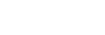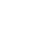8.2.4 Redox Titrations

Redox Titrations

Redox Titrations

• Iron has variable oxidation states and this characteristic can be exploited in redox titrations of Fe2+ with MnO4 or Cr2O72-
• Both the manganate(VII) ion and dichromate(VI) ions can be used as strong oxidising agents to find the amount of iron(II) ions in solution
• You need to be able to perform these separate redox titrations with Fe2+ and understand the colour changes in each example

Potassium manganate(VII) titrations

• In these redox titrations the manganate(VII) is the oxidising agent and is reduced to Mn2+(aq)
• The iron is the reducing agent and is oxidised to Fe2+(aq) and the reaction mixture must be acidified, to excess acid is added to the iron(II) ions before the reaction begins
• The choice of acid is important, as it must not react with the manganate(VII) ions, so the acid normally used is dilute sulfuric acid
• As it does not oxidise under these conditions and does not react with the manganate(VII) ions
• You could be asked why other acids are not suitable for this redox titration in the exam so make sure you understand the suitability of dilute sulfuric acid

Table explaining why other acids are not suitable for the redox titrationIndicator and end point

• Potassium permanganate acts as its own indicator, as the purple potassium permanganate solution is added to the titration flask from the burette and reacts rapidly with the Fe2+(aq)
• The burette used in this practical should be one with white numbering not black, as you would struggle to read the values for your titres against the purple colour of the potassium permanganate if black numbering was used
• The manganese(II) ions, Mn2+(aq), have a very pale pink colour but they are present in such a low concentration that the solution looks colourless
• As soon as all of the iron(II), Fe2+(aq), ions have reacted with the added manganate(VII) ions, Mn7+(aq), a pale pink tinge appears in the flask due to an excess of manganate(VII) ions, Mn7+(aq)Redox titration colour change for potassium permanganate and iron(II) ions

Worked Example

Equations

Find the stoichiometry for the reaction and complete the two half equations:

MnO4(aq) + 5e– + 8H+ (aq) → Mn2+ (aq) + 4H2O (l)

Fe2+ (aq) → Fe3+ (aq) + e

Balance the electrons:

MnO4(aq) + 5e– + 8H+ (aq) → Mn2+ (aq) + 4H2O (l)

5Fe2+ (aq) → 5Fe3+ (aq) + 5e

MnO4(aq) + 8H+ (aq) 5Fe2+ (aq) → Mn2+ (aq) + 4H2O (l) + 5Fe3+ (aq)

Potassium dichromate(VI) titrations

• Dichromate(VI) is a powerful oxidising agent when it changes from +6 oxidation state in the dichromate(VI) ion, Cr2O72-, to the +3 oxidation state in Cr3+
• The two half equations are:
• Cr2O72- (aq) + 14H+ (aq) + 6e– → 2Cr3+ (aq) + 7H2O (l)
• 6Fe2+ (aq) → 6Fe3+ (aq) + 6e
• Overall equation is:
• Cr2O72- (aq) + 14H+ (aq) + 6Fe2+ → 2Cr3+ (aq) + 6Fe3+ (aq) + 7H2O (l)

Indicator and end point

• The colour change for this titration is from orange to bluish green
• to give a more definitive and visible endpoint, the indicator sodium diphenylaminesulfonate is used
• This turns from colourless to purple at the endpoint

Exam Tip

General sequence for redox titration calculations

1. Write down the half equations for the oxidant and reductant
2. Deduce the overall equation
3. Calculate the number of moles of manganate(VII) or dichromate(VI) used
4. Calculate the ratio of moles of oxidant to moles of reductant from the overall redox equation
5. Calculate the number of moles in the sample solution of the reductant
6. Calculate the number of moles in the original solution of reductant
7. Determine either the concentration of the original solution or the percentage of reductant in a known quantity of sample

Worked Example

An iron tablet, weighing 0.960 g was dissolved in dilute sulfuric acid. A titre of 28.50 cm3 of 0.0180 mol dm-3 potassium dichromate(VI) solution was needed to reach the endpoint.

What is the percentage by mass of iron in the tablet?

• Cr2O72- (aq) + 14H+ (aq) + 6Fe2+ → 2Cr3+ (aq) + 6Fe3+ (aq) + 7H2O (l)
• 1 : 6 ratio of Cr2O72- : Fe2+
• Number of moles of Cr2O72- == 5.13 x 10-4 moles
• Moles of iron(II) = 6 x 5.13 x 10-4 = 3.078 x 10-3 moles
• Mass of iron(II) = 56.0 x 3.078 x 10-3 = 0.1724 g
• Percentage by mass =x 100  = 18.0%
CloseClose